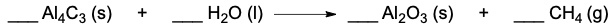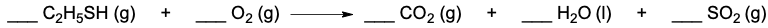Clutch Prep is now a part of Pearson
Ch.3 - Chemical ReactionsWorksheetSee all chapters

# Balancing Chemical Equations

See all sections
Sections
Empirical Formula
Molecular Formula
Combustion Analysis
Combustion Apparatus
Polyatomic Ions
Naming Ionic Compounds
Writing Ionic Compounds
Naming Ionic Hydrates
Naming Acids
Naming Molecular Compounds
Balancing Chemical Equations
Stoichiometry
Limiting Reagent
Percent Yield
Mass Percent
Functional Groups in Chemistry

Balancing Chemical Equations is the first step in future chemical calculations.

###### Balancing Chemical Equations

Concept #1: Balancing Chemical Equations

Example #1: Write the balanced equation for the following by inserting the correct coefficients in the blanks:

___ C4H10 (g) + ___ O2 (g) ___ H2O (l) + ___ CO2 (g)

Practice: Write the balanced equation for the following by inserting the correct coefficients in the blanks.Practice: Determine the total sum of the coefficients after balancing the following equation.Practice: Write the balanced chemical equation for the reaction that occurs when the gasoline additive methyl tert-butyl ether, C5H12O (l), burns in air.